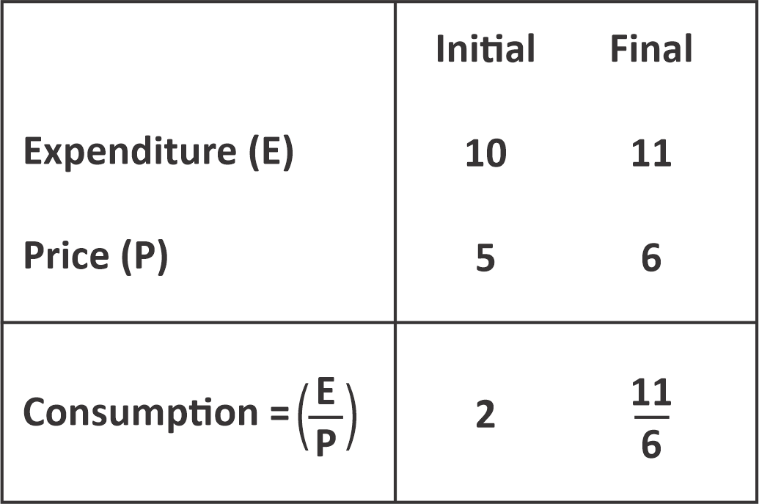Question of The Day07-09-2020

If the price of petrol increase by 20% and so does the spending limit on purchasing petrol does by 10%. By what percentage should the person reduce his consumption?

Correct Answer : b ) $$16\frac{2}{3}$$%

Explanation :

Quick Approach

We know,

20%=$$\frac{1}{5}$$and 10%=$$\frac{1}{10}$$

Let us assume the initial petrol price be 5 units and the initial expenditure be 10 units.

We know,

Expenditure = Price × consumption

$$Consumption=\dfrac{Expenditure}{Price}$$

⇒Initial consumption=$$\frac{10}{5}$$=2 unitsFrom the above figure,

% Reduction in consumption=$$\frac{initial-final}{ initial}×100=\frac{2-\frac{11}{6}}{2}×100$$=$$8\frac{1}{2}$$%

Hence, (b) is the correct answer.

Basic Approach

Let us assume the initial price of petrol be ₹ x/liter

Thus, the final price of petrol=x+20% of x=x+$$\frac{1}{5}$$x=$$\frac{6}{5}$$x

Let us assume the initial expenditure on petrol be ₹ y

Thus, the final expenditure on petrol=y+10% of y=y+$$\frac{1}{10}$$y=$$\frac{11}{10}$$y

We know,

Expenditure = Price × consumption

Thus, initial consumption=$$\frac{initial \ expenditure}{initial \ price}$$=$$\frac{y}{x}$$

⇒Final consumption=$$\frac{Final \ expenditure} {Final \ price}$$=$$\frac{\frac{11}{10}y}{\frac{6}{5}x}$$=$$\frac{11y}{12x}$$

% Reduction in consumption=$$\frac{Initial-final}{initial}×100$$=$$\frac{\frac{y}{x}-\frac{11y}{12x}}{\frac{y}{x}}×100$$=$$8\frac{1}{2}$$%

Hence, (b) is the correct answer.

Such type of question is asked in various government exams like SSC CGL, SSC MTS, SSC CPO, SSC CHSL, RRB JE, RRB NTPC, RRB GROUP D, RRB OFFICER SCALE-I, IBPS PO, IBPS SO, RRB Office Assistant, IBPS Clerk, RBI Assistant, IBPS RRB OFFICER SCALE 2&3, UPSC CDS, UPSC NDA, UP SI, etc.

Read Daily Current Affairs, Banking Awareness, Hindi Current Affairs, Word of the Day, and attempt free mock tests at PendulumEdu and boost your preparation for the actual exam.0